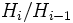# Order of group is product of orders of composition factors

## Statement

Suppose$G$ is a group of finite composition length with a composition series:$\{ e \} = H_0 \le H_1 \le H_2 \le \dots \le H_n = G$

In particular, this means that each$H_{i-1}$ is a normal subgroup of$H_i$ and the quotient group$H_i/H_{i-1}$ is a simple group. Note that, by the Jordan-Holder theorem, we know that, counting repetitions and up to isomorphism and re-ordering, the collection of groups$H_i/H_{i-1}$ is uniquely determined by$G$ and independent of the choice of composition series. These groups are called the composition factors.

We have the following:$|G| = \prod_{i=1}^n |H_i/H_{i-1}|$

Another way of putting this is that the order of the group is the product of the orders of the composition factors (each composition factor multiplied as many times as its multiplicity).

Note that:

•$G$ is a finite group if and only if all the composition factors are finite groups, and in this case the multiplication is the usual multiplication of natural numbers.
•$G$ is infinite if and only if at least one of the composition factors is infinite. In this case, we use cardinal multiplication to determine the order. Assuming the axiom of choice, the order of$G$ is the maximum of the orders of the composition factors. For more, see cardinal multiplication.

## Related facts

### Applications

• One of the definitions of finite solvable group is that all its composition factors are abelian, which is equivalent to saying that all its composition factors are cyclic of prime order. The constraint on the product of orders now tells us that, if the order of a finite solvable group is$n = p_1^{k_1}p_2^{k_2} \dots p_r^{k_r}$ for distinct primes$p_i$, then the composition factors must be as follows:$k_i$ composition factors isomorphic to the group of order$p_i$ for$1 \le i \le r$. Thus, there is a total of$k_1 + k_2 + \dots + k_r$ composition factors counting multiplicities. Note also that the multiset of composition factors is the same for any two finite solvable groups of the same order.
• Classification of possible multisets of composition factors for groups of a given order: Suppose we are given a natural number$n$ and are asked to determine all the possibilities for the multiset of composition factors (i.e., the isomorphism classes of composition factors with multiplicities) of groups of order$n$. We can sketch a procedure to do this: first, find the prime factorization of$n$. Then, find all the simple non-abelian groups of orders dividing$n$. Find all possible multisets of these simple non-abelian groups for which the product of orders in the multiset divides$n$. For each such multiset, complete it by choosing groups of prime order for the remaining composition factors. These are precisely the possible multisets.

## Facts used

1. Order of group is product of orders of successive quotient groups of subnormal series

## Proof

The proof is direct from Fact (1), once we note that a composition series is a special type of subnormal series.